# The upside-down pendulum

Balancing a pencil on the tip of your finger is difficult, isn't it? But to stabilize a rigid pendulum in the upside-down position is easy! You don't need sophisticated controlling technology. You only need a simple and fast motor moving the suspension point of the pendulum periodically up and down! If you don't believe me, try it in the lab!

How can this stabilization effect be understood? Starting from the equation of motion of a vertically driven pendulum

 (1)and applying the Kapiza method is the easiest way to understand this effect. This method was invented by the russian physicist P.L. Kapiza (1894-1984) in order to treat analytically systems with a natural time scale much larger than the time scale of driving. It is only an approximative method, but it works quite well in cases where these time scales are of different order of magnitude. The method starts with the ansatz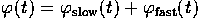.

whereslow is the slowly variing part andfast the fast one. That is, averaged over the fast time scale yields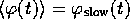. Putting this ansatz into the equation of motion yields two equation, one for the slow time scale:

 (2)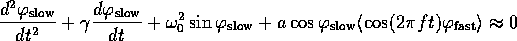and one for the fast time scale:

 (3)Two approximations are made. First, everything is linearized infast, assuming that the fast oscillations are small. Here. In the last equation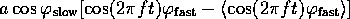has been neglected. This is justified as long as both time scales are well separated. The time dependence ofslow can be neglected in the equation of motion forfast. Thus,slow can be treated as a constant. Therefore, eq. (3) can be easily solved:Multiply this with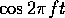and average over the fast time scale, you will get an equation of motion forslow alone:

 (4)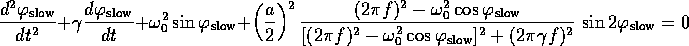This is an equation of motion for the slowly variing part of the motion. The averaged product of the fast oscillation of the driving and the fast oscillation of the system leads to an effective force on the pendulum. In the upside-down position it is a restoring force. For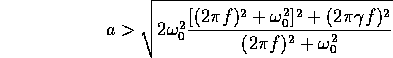it is stronger than the repelling force and the upside-down pendulum is stabilized. That is, the solutionslow =180° is a stable solution of (4) and in the framework of the Kapiza method also of (1). The stabilization of the upside-down pendulum is accompanied by a subcritical pitchfork bifurcation of (4).

Dynamical stabilization is applied in a so-called Paul trap where a single ion can be catched. The ion sits in an equilibrium point of a static quadrupole field. This equilibrium point is unstable as any equilibrium point of a static electrical field. But a rapidly oscillating electrical field (typical frequency 1Mhz) is able to turn it into a stable equilibrium point.

 QUESTIONS worth to think about: Apply the Kapiza method to the horizontally driven pendulum. How does it behave for large driving frequencies? Show that the down-hanging equlibrium becomes unstable if the driving amplitude exceeds a critical value. What kind of bifurcation occurs?

© 1998 Franz-Josef Elmer,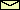Franz-Josef doht Elmer aht unibas doht ch, last modified Friday, July 24, 1998.